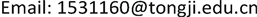1上海轨道交通十三号线发展有限公司，上海

2同济大学经济与管理学院，上海1. 引言

2. PIP的功能与设计

2.1. PIP的功能

2.2. PIP的设计

PIP系统分为用户管理、用户信息交流、公共信息发布、项目信息沟通、项目文档管理、日常管理工具、系统内容管理七大模块。

3. PIP综合评价指标体系

1) 整体视觉效果。

2) 技术功能指标。

Comprehensive evaluation index system of PI

RIA外观a2

3) 标准性能指标。

4) 成本指标。

4. 基于相对熵的多属性决策综合评价法4.1. 现行常用评价方法简述

4.2. 相对熵排序方法的具体步骤

y i j = x i j x j max (j为效益型属性) (1)

y i j = x j min x i j (j为成本型属性) (2)

x j max = max { α 1 j , α 2 j , ⋯ , α m j } , j = 1 , ⋯ , n (3)

x j min = min { α 1 j , α 2 j , ⋯ , α m j } , j = 1 , ⋯ , n (4)

ω j ( 1 ) = d j ∑ j = 1 n d j (5)

d j = 1 − E j , E j = − ∑ i = 1 m p i j ln p i j ln m (6)

p i j = y i j ∑ i = 1 m y i j (7)

W * = ( ω 1 * , ⋯ , ω j * , ⋯ , ω n * ) (8)

S i * = ∑ j = 1 n { z * log z * z i j + ( 1 − z * ) log ( 1 − z * ) ( 1 − z i j ) } (11)

S i − = ∑ j = 1 n { z − log z − z i j + ( 1 − z − ) log ( 1 − z − ) ( 1 − z i j ) } (12)

C i * = S i − S i * + S i − , i ∈ M (13)

N i * = S i − S i * , i ∈ M (14)

4.3. 案例分析

Final scoring table of PI

Aa144333
a244333
Bb121243
b234322
b321243
b434322
b534322
b632322
b722141
b821243
b932322
b1021243
b1134322
Cc122141
c221243
c334322
c432322
c534322
Dd144333
d221243
d321243

Y = [ 1.00 0.50 0.75 0.50 1.00 0.33 1.00 0.25 1.00 0.50 0.67 0.50 0.75 0.50 0.75 0.25 1.00 0.50 0.75 1.00 0.50 1.00 0.67 1.00 0.75 0.75 0.50 0.25 0.67 0.25 ] (15)

ω j * = ( 0.1185 , 0.2185 , 0.1357 , 0.24025 , 0.09375 , 0.1683 ) (16)

z = [ 0.1185 0.1093 0.1081 0.1201 0.0938 0.0555 0.1185 0.0546 0.1357 0.1201 0.0628 0.0842 0.0889 0.1093 0.1018 0.0601 0.0938 0.0842 0.0889 0.2185 0.0678 0.2402 0.0628 0.1683 0.0889 0.1639 0.0678 0.0601 0.0628 0.0421 ] (17)

5. 结语

1) 目前国内外许多对于PIP的研究都停留在其功能、应用以及与管理信息系统的区别上，而本文重点研究了城市地铁项目中PIP的评价系统，这对于PIP的发展具有重要意义，同时也为以后的研究提供了参考。

2) 本文提出了一套较为完整、科学的评价指标。不仅考虑了传统PIP的评价指标，也考虑了城市地铁项目中PIP的特殊性，从而建立了一套适用于评价地铁项目PIP的指标。

3) 本文采用相对熵排序法，将传统TOPSIS方法进行改进，使得不同地铁项目PIP的评价结果直观清楚，具有一定的推广意义。